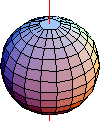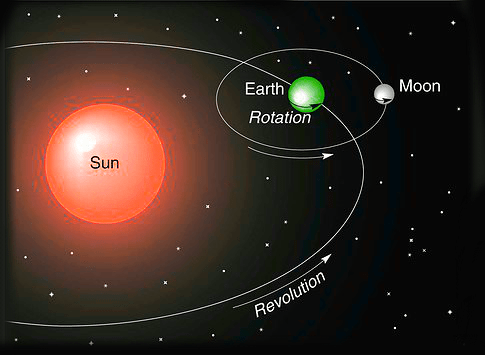# Rotation and Revolution

## Earth Rotation and Revolution

Introduction:

At the outset, these two words are used synonymously. That is incorrect. The two terms, Rotation and Revolution refer to two totally different phenomena.

Everybody that we see, has its own center of mass. When a body has a circular motion about its axis and when the axis passes through its center of mass, it is called as Rotation. A figure of a sphere shown below is an example of rotation. The red line is the axis line which passes through its center of mass.We all have seen a top spinning about its axis, this is another example of rotation. Rotation can be better understood as spinning. Now, when you look at the term revolution, the difference is about the point around which the circular motion happens. We all know that the planets go around the sun. The sun here is the point of reference but it is external to the planet, and the planets move around this external reference point. Such a circular motion about a reference point external to a body is called Revolution. Generally in specific fields like astronomy, when the term revolution is used, they call it as orbital revolution.

Units of Measurement:

Though both are circular motions, the units used to measure them are not the same. When it comes to measuring rotation, the unit of measurement is angular frequency measured in radians/second (rad/sec) or turns/unit time. The rate of change of this angular frequency, i.e., the change of this frequency with reference to a given time rate is called as angular acceleration (Rad/sec2). This is similar to Velocity and acceleration in linear motion, where Velocity is m/s and acceleration is m/s2.
Revolution on the other hand is the time taken to go around a particular external point. When we talk about engines, it is mentioned as RPM. That is why we use the terms like Orbital Revolution of earth around sun, while it rotates about its own axis. Ex: earth takes 365 days to go around the sun.

Rotational speed has two components. One of them is the linear component called tangential speed. It is the distance covered in unit of time. While in rotation if you released the object it will fly at a tangent from the point of release at a specific speed.

The other component is the rotational speed. It is the number of rotations per unit time. For example: Many of us have seen circular plates rotating, or even the LP music records of yesteryears. Some of these records rotate at specific speeds like 33 revolutions per minute. The rotational speed of all points in this plate are the same, but if you look at the linear speed they will be different. The point closer to the center of the circle will have a slightly lesser linear speed when compared to the point closer to the circumference. So two points on a circle can have same rotational speed but different linear speed as linear speed is dependent on the radial distance. The distance travelled by an object in one rotation is equal to its circumference.

Also, a rotating body has its kinetic energy and potential energy. The angular kinetic energy of a rotating body is a part of its total kinetic energy. A rotating body also its rotational potential energy by virtue of its position during the rotation. The sum of the KE and PE is the total rotational energy of the object. However, the total energy is always conserved.

When we travel in vehicles and while taking a turn we all have experienced being thrown to the outside depending on the turn that we take. This force is a reaction to what is called the centripetal force and is given by the formula MV2/R where M is the Mass, V is the Tangential Velocity and R is the radius of the turn. The centripetal force is the force that ensures that a body follows or takes a curved path. Isaac Newton described this as “the force by which bodies are drawn or impelled, or in any way tend, towards a point as to a centre”. For astronomical orbits, it is gravity that provides the centripetal force. The acceleration caused in such rotational motions is called Centripetal acceleration and is given by V2/R. The force that acts against this centripetal force is called the Centrifugal force. This is sometimes also called as the “Fictitious” force. It is directed away from the rotational axis. If a moving car enters into a curve that is bending to the left, then any passenger sitting inside the vehicle will experience a force that seems to be pulling him or her towards the right. This is the fictitious centrifugal force.

There are so many amusement rides which we would have experienced. For ex: the Ferris Wheel. It has a horizontal central axis and a parallel axis for each cabin, where the rotation is in opposite direction either because of mechanical operation or gravity. Because of this, at any time, the cabin remains upright and not rotated, but just translated. In case of rides like the roller coaster with inversions, the rotation about the horizontal axis may comprise of just one cycle or more than one cycle and in this case the inertia keeps people in the chairs or seats. In sports, when we watch a tennis match, there is topspin and backspin.
Spin or rotation plays a significant role in such cases causing direction to change after bounce or reducing the speed etc:
In Revolution, as we discussed earlier the axis of motion is located outside the object. A completion of a circular trip around a particular point is called a revolution. In this case the axis is external to the body. Further in revolution there is change in position of the body but in rotation there is no change in position. The distance travelled in one rotation and distance travelled in one rotation need not be the same. However, in the case of the moon around the earth, it is the same that is why we see the same face of the moon always!

Try solving this problem:

Find the distance that earth will travel in one day in its orbit around the sun. Assume a year of 365 days and that the path around the sun is a circle of radius 93 million miles. Compare this distance with the distance travelled in earth’s one rotation given that radius of earth is 6371kms. The clue for the second part is to find the circumference of the earth.
For the first part of the problem it is 2x3.14x9300000/365 = 160010 miles per day in its orbit around the sun.Talking of rotational motion, let us understand another concept closer to it. That is Torque. Do you remember situations where you try to loosen a nut with a spanner and apply increasing force and not being able to remove it? If you get a slightly longer spanner, it’s a bit easier and you find that you are able to do the work easily. Torque essentially is the force that makes an object turn around its axis. This force is dependent on the distance from the centre. This is measured in Nm (Newton Meter). Essentially this is the force required to overcome rotational inertia. In linear motion we have the force of inertia to overcome which we apply a linear force. Once the applied force is greater than the inertia the body moves. Similarly when the torque applied is greater than the rotational inertia, it is possible to rotate the body.

Planetary motion follow the laws of revolution. These are mainly Kepler’s laws of planetary motion. These were derived by Johannes Kepler, a German astronomer. Also, influencing the motion of the cosmic bodies are the gravitational laws of Sir Isaac Newton. The effects of rotation and revolution are clearly felt by these motions on earth. Rotation of the earth about its own axis causes the night and day we perceive.

Some daily uses of forces of rotation:

Washing machine dryer: The dryer is nothing but a basket spinner. It is a cylinder with many holes on its sides. When it spins (rotates) at high speeds, the water is thrown out through the holes.

Cream Separator:
When we need to remove or separate the cream from the milk, we use these machines. These are similar to centrifuge tubes.
When the milk with cream is put into the bowls and made to rotate at very high speeds, the heavier content in the milk moves outwards. The lighter or less heavier contents move towards the centre. The dense skimmed milk collects towards the outside and the lighter cream gets collected towards the center.

In conclusion, it is to be clearly understood and remembered that rotation and revolution are not the same. They are different and their effects are also different. The laws governing rotation and revolution and the forces thereof are also not the same.